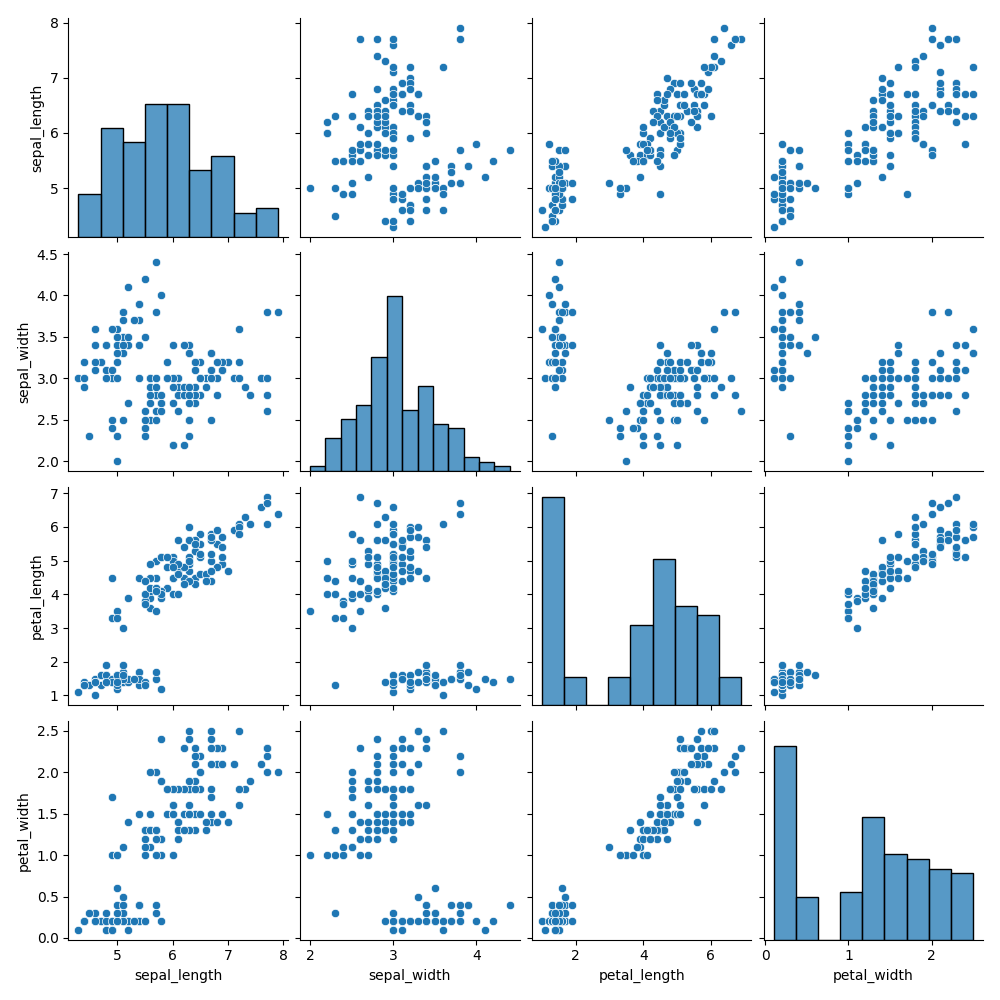Course Content

# Ultimate Visualization with Python

1. Matplotlib Introduction

2. Creating Commonly Used Plots

4. More Statistical Plots

5. Plotting with Seaborn

Ultimate Visualization with Python

##Pair Plot

Pair plot is used to plot a pairwise relationship between the numeric variables in a dataset. It is quite similar to a joint plot, however, it is not limited to only two variables. In fact, a pair plot creates an `NxN` grid of the `Axes` objects (multiple subplots) where `N` is the number of numeric variables (numeric columns in a `DataFrame`).

Let’s have a look at an example of such plot:### Pair Plot Description

As you can see, for each column x-axis is shared among all the plots in the columns, a certain single variable lies on the x-axis. The same goes for the rows where the y-axis is shared among all the plots in the row. Diagonal plots are histograms by default, since they show the distribution of a single variable (univariate marginal distribution), and the other plots are scatter plots.

### Creating a Pair Plot

Creating a pair plot with `seaborn` comes down to calling its `pairplot()` function. Its most important and the only required parameter is `data` which should be a `DataFrame` object. Here is an example for you:Here `iris_df` is the `DataFrame` we pass in the `pairplot()` function and everything works just fine. `height` and `aspect` parameters just specify the height and width (`height * aspect`) of each facet (side) in inches.

### Hue

Another parameter which is worth mentioning is `hue` which specifies the variable (column name) in `data` to map plot aspects to different colors or even create separate plots (on one `Axes`) for each of its values.

Here is an example to make things clear:You can instantly spot the difference here. First of all, the data points on each scatter plot are colored according to the species they belong to (the respective value in the `’species’` column). Diagonal plots are now KDE plots (a separate one for each of the species) instead of the histograms.

As a matter of fact, when dealing with a classification problem it often makes sense to create a pair plot with the `hue` parameter set to the target variable (categorical variable we want to predict).

### Changing Plot Kinds

You can also set other plots instead of the scatter plots and set other diagonal plots. The parameters `kind` (`'scatter'` is its default value) and `diag_kind` (`'auto'` is its default value, so its kind is based on the presence of the `hue` parameter) respectively are used for this purpose.

Let’s now modify our example:`'scatter'`, `'kde'`, `'hist'`, `'reg'` are possible values for the `kind` parameter.

`diag_kind` can be set to one of the following values:

• `'auto'`;
• `'hist'`;
• `'kde'`
• `None`.

Everything is similar to the `jointplot()` function in this regard.

More on the `pairplot()` function in its documentation.

1. Use the correct function to create a pair plot.
2. Set the data for the plot to be `penguins_df` via the first argument.
3. Set `'sex'` as the column which will map the plot aspects to different colors via specifying the second argument.
4. Set the non-diagonal plots to have a regression line (`'reg'`) via specifying the third argument.
5. Set `height` to `2`.
6. Set `aspect` to `0.8`.

It may take a few minutes to verify the solution.

Everything was clear?

Section 5. Chapter 6